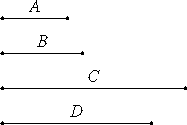# Proposition 18

Given two numbers, to investigate whether it is possible to find a third proportional to them.

Let A and B be the given two numbers. It is required to investigate whether it is possible to find a third proportional to them.

IX.16

Now A and B are either relatively prime or not. And, if they are relatively prime, it was proved that it is impossible to find a third proportional to them.

Next, let A and B not be relatively prime, and let B multiplied by itself make C. Then A either measures C or does not measure it.

First, let it measure it according to D, therefore A multiplied by D makes C. But, further, B multiplied by itself makes C, therefore the product of A and D equals the square on B.VII.19

Therefore A is to B as B is to D, therefore a third proportional number D has been found to A and B.

Next, let A not measure C.

I say that it is impossible to find a third proportional number to A and B.

If possible, let D be such third proportional. Then the product of A and D equals the square on B. But the square on B is C, therefore the product of A and D equals C.

Hence A multiplied by D makes C, therefore A measures C according to D.

But, by hypothesis, it also does not measure it, which is absurd.

Therefore it is not possible to find a third proportional number to A and B when A does not measure C.

Q.E.D.

## Guide

Note that a third proportional d to a and b has to satisfy a : b = b : d, so d would have to be b2/a. So the third proportional exists when a divides b2. This conclusion is just what Euclid discovers in this proposition.